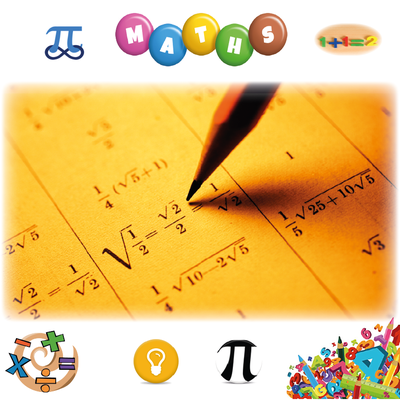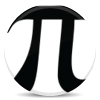Learn ﻿﻿﻿Mathematics

An easy way to learn Mathematics online for free. Learn Maths Basics & Prealgebra; Geometry, Algebra & Trigonometry; Precalculus, Calculus & much more through this very simple course. Multiple Choice Tests. Thanks to Mem creators, Contributors & Users.
64.5k người học thời gian trung bình 209h100 x 100  Multiplication Table

30k 81hMaths GCSE

16.4k 50mMaths

Learn Times Tables

16.1k 1hMultiplication Tables

13.6k 2hMaths

Learn ﻿﻿﻿﻿﻿﻿﻿Statistics

12k 17h'Basic Trigonometric Identities'

11.9k 35mProbability Theory and Statistics (Introduction)

6.74k 7hA level Edexcel Maths and Further Maths

6.15k 2h100 Perfect Squares

6.12k 2h150 Digits of Pi

5.42k 37mEdexcel D1 Definitions

4.83k 25mMaths

General Maths (Methods) CAS VCE - Trigonometry

3.72k 21mMaths

1 - 144 Times Tables! Multiplication and division

3.56k 5hMaths

Conversions

3.23k 19m3.1k 22m